Question

# ZilIDiffEQ9 4.9.021. 4. Solve the given initial-value problem.

ZilIDiffEQ9 4.9.021. 4.

Solve the given initial-value problem.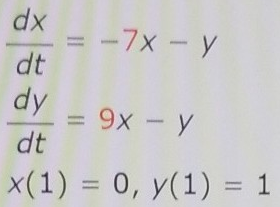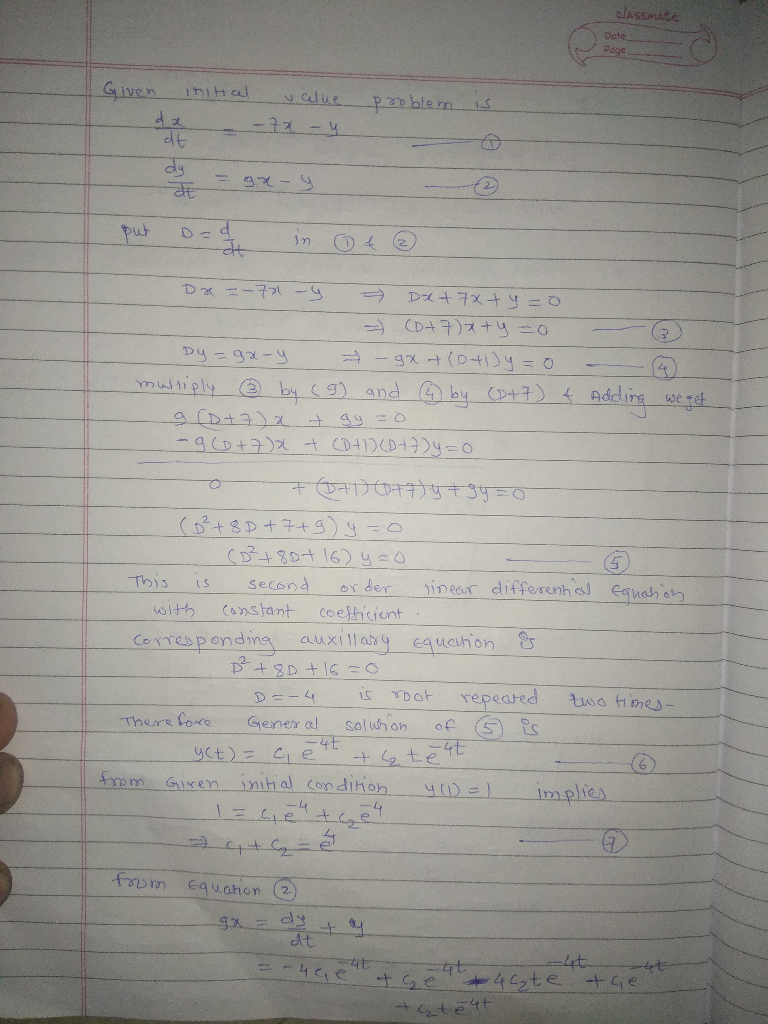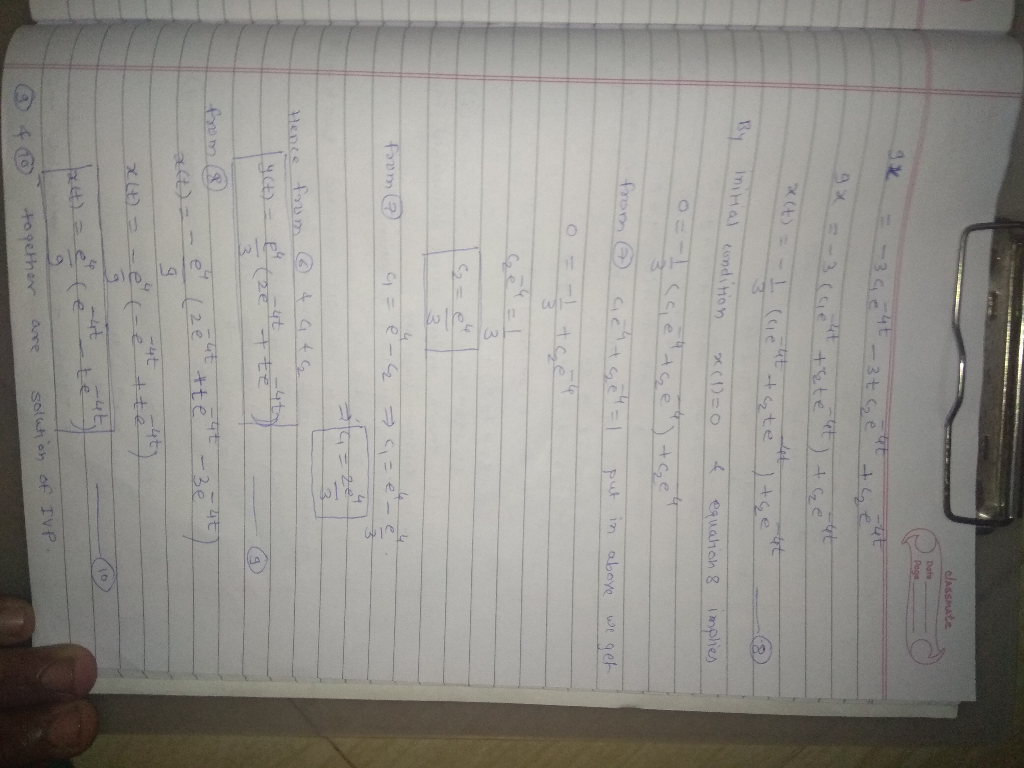#### Earn Coins

Coins can be redeemed for fabulous gifts.

Similar Homework Help Questions
• ### Solve initial value problem using Laplace transform Problem 4 Solve the initial value problems given below...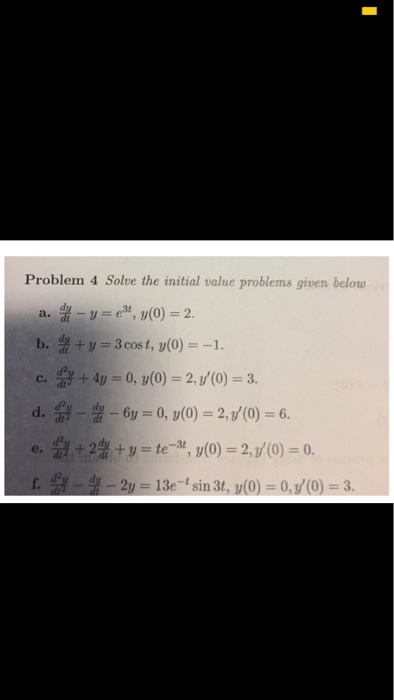Solve initial value problem using Laplace transform Problem 4 Solve the initial value problems given below --ез, y(0) 2. a. b. f ty 3 cos t, y(0)-

• ### solve the given initial-value problem For Problems 37-40, solve the given initial-value problem. 38. y" =...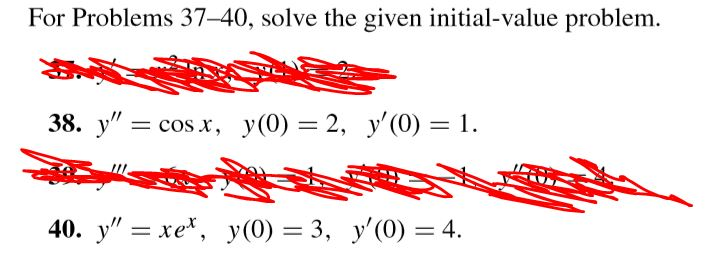solve the given initial-value problem For Problems 37-40, solve the given initial-value problem. 38. y" = cos x, y(0) = 2, y'(0) = 1. 40. y” = xe", y(0) = 3, y'(0) = 4.

• ### Solve the given initial-value problem. 8-1), x(0)=( -4 8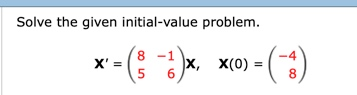Solve the given initial-value problem. 8-1), x(0)=( -4 8 Solve the given initial-value problem. 8-1), x(0)=( -4 8

• ### Solve the given initial value problem. Thank you! Solve the given initial value problem. y''' +...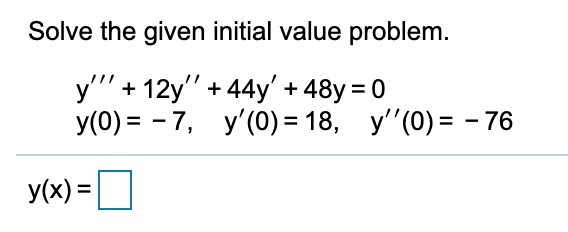Solve the given initial value problem. Thank you! Solve the given initial value problem. y''' + 12y'' +44y' +48y = 0 y(O)= -7, y'(0) = 18, y''(0) = - 76 y(x) =

• ### 2. Solve the initial value problem for the given differential equation. 2. Solve the initial value problem for the given differential equation.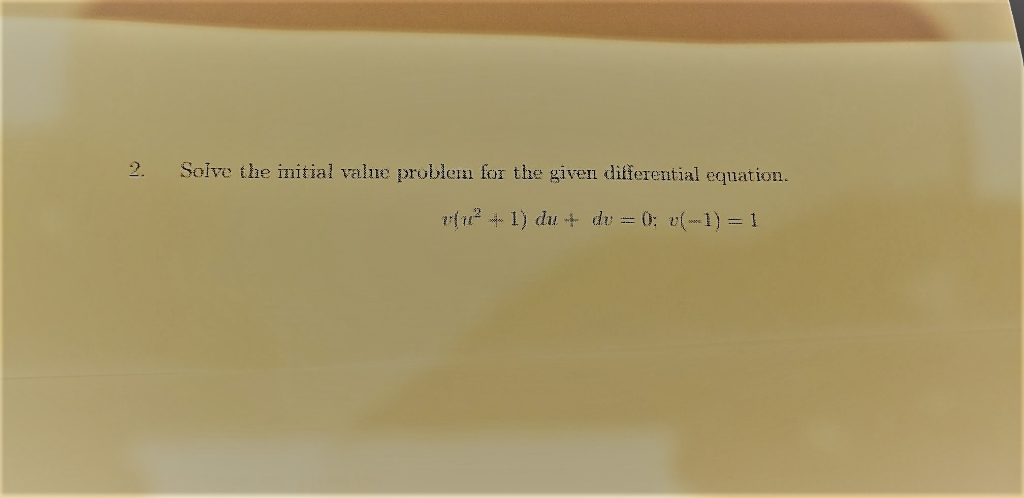2. Solve the initial value problem for the given differential equation. 2. Solve the initial value problem for the given differential equation.

• ### Solve the given initial-value problem. Solve the given initial-value problem. 1 X' = 0 0 1...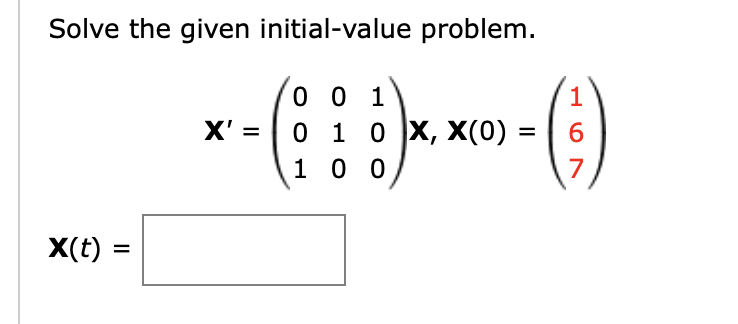Solve the given initial-value problem. Solve the given initial-value problem. 1 X' = 0 0 1 0 1 0 X, X(0) = 1 0 0 6 7 X(t)

• ### Solve the given initial-value problem.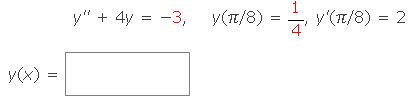Solve the given initial-value problem.

• ### Solve the given initial-value problem.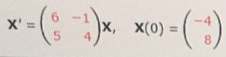Solve the given initial-value problem.

• ### Solve the given symbolic initial value problem and sketch a graph of the solution y" +...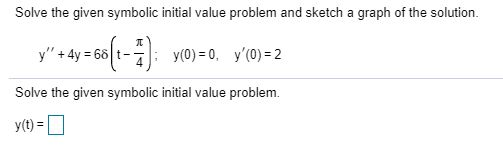Solve the given symbolic initial value problem and sketch a graph of the solution y" + 2y =60(1-4): y0)=0, y(0) = 2 Solve the given symbolic initial value problem. y(t) =

• ### ZillDiffEQ9 8.2.048 Solve the given initial-value problem.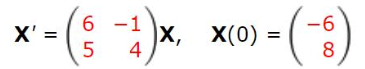ZillDiffEQ9 8.2.048 Solve the given initial-value problem.